# Calculators

Can you remember Avogadro's constant to six decimal places? Can you figure out the square root of 747 in less than a second? Can you add up hundreds of numbers, one after another, without ever making a mistake? Pocket calculators can do all these things and more using tiny electronic switches called transistors. Let's take a peek inside a calculator and find out how it works!

Photo: This Casio fx-570 calculator has given me sterling service since 1984 and is still going strong today. In case you're wondering, Avogadro's constant (one of many constants stored in this calculator and available at the touch of a button) used to be quoted as 6.022045 × 1023 (since 2011, newer sources have given a more accurately calculated value of 6.022141 × 1023).

## What is a calculator?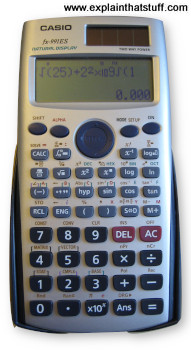Photo: My newer Casio Calculator, an fx-991ES, has a much larger "natural display" that can show entire equations and even perform calculus! The larger dark gray keys at the bottom are the numbers and the main "operators" (+, −, ×, ÷, = etc). The lighter gray keys above them carry out a whole range of scientific calculations with a single button click. The brown-colored square in the extreme top right is a solar cell that powers the machine along with a small button battery.

Our brains are amazingly versatile, but we find it hard to calculate in our heads because they can store only so many numbers. According to a famous bit of 1950s research by psychologist George Miller, we can remember typically 5–9 digits (or, as Miller put it: "the magical number seven, plus or minus two") before our brains start to ache and forget. That's why people have been using aids to help them calculate since ancient times. Indeed, the word calculator comes from the Latin calculare, which means to count up using stones.Photo: A Burroughs Mechanical calculator from the early-20th century. You enter the numbers you want to work with using the nine columns of octagonal keys at the top, crank the handle, and read the result in the little "windows" at the bottom. Photo courtesy of National Institute of Standards and Technology Digital Collections, Gaithersburg, MD 20899.

Mechanical calculators (ones made from gears and levers) were in widespread use from the late-19th to the late-20th century. That's when the first affordable, pocket, electronic calculators started to appear, thanks to the development of silicon microchips in the late 1960s and early 1970s.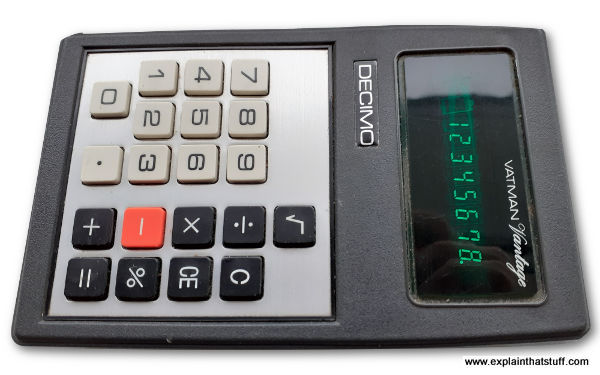Photo: This is what calculators looked like in the 1970s. Note the very basic 8-digit green display (it's called a vacuum fluorescent display) and the relatively small number of mathematical functions (all you could really do was +, −, ×, ÷, square roots, and percentages). What you can't see from this photo is how thick and chunky this calculator was and how big its batteries were. Modern calculators are far more advanced, much cheaper, and use a fraction as much battery power.

Modern calculators have much in common with computers: they share much of the same history and work in a similar way, but there's one crucial difference: a calculator is an entirely human-operated machine for processing math, whereas a computer can be programmed to operate itself and do a whole range of more general-purpose jobs. In short, a computer is programmable and a calculator is not. (A programmable calculator sits somewhere between the two: you can program it, but only to do relatively simple mathematical calculations.)

## What's inside a calculator?

If you'd taken apart a 19th-century calculator, you'd have found hundreds of parts inside: lots of precision gears, axles, rods, and levers, greased to high heaven, and clicking and whirring away every time you keyed in a number. But take apart a modern electronic calculator (I just can't resist undoing a screw when I see one!) and you might be disappointed at how little you find. I don't recommend you do this with your brand-new school calculator if you want to stay on speaking terms with your parents, so I've saved you the bother. Here's what you'll find inside: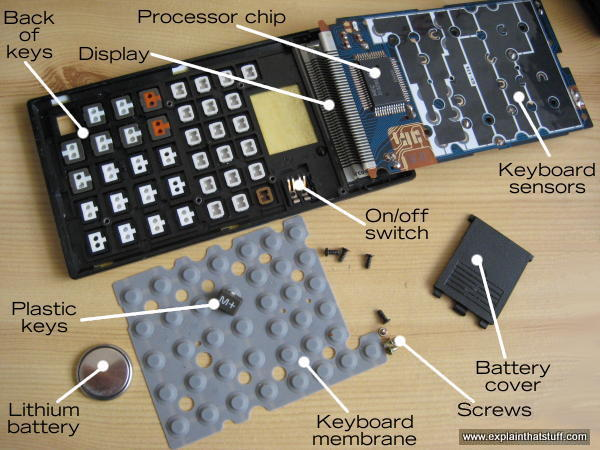Caption: Inside the fx-570, which is face-down here. We're effectively looking up into the machine from below.
Don't worry, I managed to put it all back together again just fine!

• Input: Keyboard: About 40 tiny plastic keys with a rubber membrane underneath and a touch-sensitive circuit underneath that.
• Processor: A microchip that does all the hard work. This does the same job as all the hundreds of gears in an early calculator.
• Output: A liquid crystal display (LCD) for showing you the numbers you type in and the results of your calculations.
• Power source: A long-life battery (mine has a thin lithium "button" cell that lasts several years). Some calculators also have a solar cell to provide free power in the daylight.

## What happens when you press a key?

Press down on one of the number keys on your calculator and a series of things will happen in quick succession:

1. As you press on the hard plastic, you compress the rubber membrane underneath it. This is a kind of a miniature trampoline that has a small rubber button positioned directly underneath each key and a hollow space underneath that. When you press a key, you squash flat the rubber button on the membrane directly underneath it.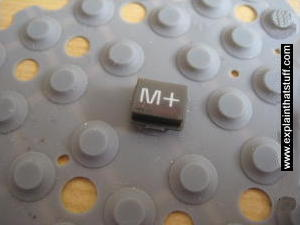Photo: The keyboard membrane. I've left one of the keys on the membrane to give you an idea of the scale. There's one rubber button directly beneath each key. Read more in our article about computer keyboards.

2. The rubber button pushes down making an electrical contact between two layers in the keyboard sensor underneath and the keyboard circuit detects this.
3. The processor chip figures out which key you have pressed.
4. A circuit in the processor chip activates the appropriate segments on the display corresponding to the number you've pressed.
5. If you press more numbers, the processor chip will show them up on the display as well—and it will keep doing this until you press one of the operations keys (such as +, −, ×, ÷) to make it do something different. Suppose you press the + key. The calculator will store the number you just entered in a small memory called a register. Then it will wipe the display and wait for you to enter another number. As you enter this second number, the processor chip will display it digit-by-digit as before and store it in another register. Finally, when you hit the = key, the calculator will add the contents of the two registers together and display the result. There's a little more to it than that—and I'll go into a few more details down below.

## How does the display work?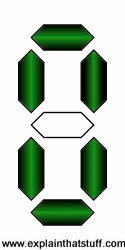Artwork: A seven-segment display can show all the numbers from 0-9.

You're probably used to the idea that your computer screen makes letters and numbers using a tiny grid of dots called pixels. Early computers used just a few pixels and looked very dotty and grainy, but a modern LCD screen uses millions of pixels and is almost as clear and sharp as a printed book. Calculators, however, remain stuck in the dark ages—or the early 1970s, to be precise. Look closely at the digits on a calculator and you'll see each one is made from a different pattern of seven bars or segments. The processor chip knows it can display any of the numbers 0–9 by activating a different combination of these seven segments. It can't easily display letters, though some scientific calculators (more advanced electronic calculators with lots of built into mathematical and scientific formulae) do have a go.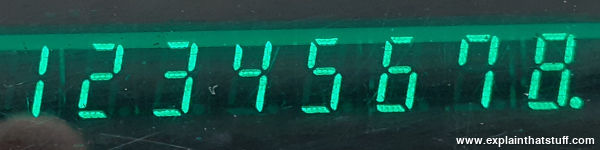Photo: Look closely at the green digits on this display and you can see that each one is made by lighting up two or more of seven segments. This is a closeup of the green vacuum fluorescent display in the 1970s calculator shown up above.

## How does a calculator add two numbers together?

So far we've had a very simple look at what's going on inside a calculator, but we've not actually got to the heart of how it takes two numbers and adds them to make a third one. For those of you who'd like a bit more detail, here's a slightly more technical explanation of how that happens. In short, it involves representing the decimal numbers we use in a different format called binary and comparing them with electrical circuits known as logic gates.

### Representing numbers in binary

Humans work on numbers in decimal format (the numbers 0–9) largely, it's believed, because we have ten fingers and toes to count with. But the numbers we use to write out amounts of things are arbitrary. Let's say you have a pile of coins and you want to tell me how wealthy you are. You can point to the pile, I can look at it, and if I see a lot of coins I'll conclude you're rich. But what if I'm not there to look at the pile? Then you can use a symbol to represent the coins—and that's what a number is: a symbol that indicates an amount. If there were nineteen coins, you could use the two symbols "1" and "9" written together: 19. Taken together, that means 1 × 10 plus 9 × 1 = 19. That's how decimal works using a system of 10 symbols. But you could use other symbols too.

For the last century or so, computers and calculators have been built from a variety of switching devices that can either be in one position or another. Just like a light-switch, they're either "on" or "off." For that reason, computers and calculators store and process numbers using what's called binary code, which uses just two symbols (0 and 1) to represent any number. So in binary code, the number 19 is written 10011, which means (1 × 16) + (0 × 8) + (0 × 4) + (1 × 2) + (1 × 1) = 19. The beauty of binary is that you can represent any decimal number with a series of switches that are either on or off—perfect for a calculator or a computer—like this: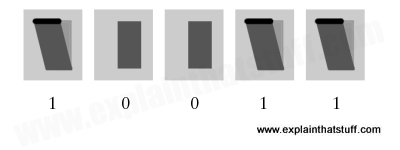Artwork: How to represent the binary number 19 inside a calculator or a computer using five switches. Three are pressed down (switched on) and two are left as they are (switched off), indicating the binary number 10011, which is equal to 19 in decimal.

### Converting decimal to binary

The first thing your calculator has to do is convert the decimal numbers you input to binary numbers it can work with, and it does that using a (fairly) simple circuit called a BCD (binary coded decimal) encoder. It's simpler than it sounds—and the animation below shows how it works for the numbers 1–9. There are 10 "input" keys (I've omitted zero) wired to four output lines. Each input is wired in such a way that it triggers one or more of the outputs, so the conversion process effectively happens through the pattern of the wiring. For example, key 1 triggers just the line on the right, giving us an output of 0001 in binary, while key 7 triggers three of the four lines giving us 0111 in binary (4 + 2 + 1).Animation: How a calculator's BCD encoder converts decimal input from the keyboard into binary output. The output lines are triggered by OR gates (described below) connected to the input lines, so each output line triggers if one OR more of the input lines connected to it send a current.

### Using logic gates with binary

Let's say you want to do the sum 3 + 2 = 5.

A calculator tackles a problem like this by turning the two numbers into binary, giving 11 (which is 3 in binary = 1 × 2 + 1 × 1) plus 10 (2 in binary = 1 × 2 + 0 × 1) makes 101 (5 in binary = 1 × 4 + 0 × 2 + 1 × 1). How does the calculator do the actual sum? It uses logic gates to compare the pattern of switches that are active and come up with a new pattern of switches instead.

A logic gate is really just a simple electrical circuit that compares two numbers (inputs) and produces a third number (an output) depending on the values of the original numbers. There are four very common types of logic gates called OR, AND, NOT, and XOR. An OR gate has two inputs (each of which can be either 0 or 1) and it produces an output of 1 if either of the inputs (or both) is 1; it produces a zero otherwise. An AND gate also has two inputs, but it produces an output of 1 only if both inputs are 1. A NOT gate has a single input and reverses it to make an output. So if you feed it a zero, it produces a 1 (and vice-versa). An XOR gate gives the same output as an OR gate, but (unlike an OR gate) switches off if both its inputs are one.

Now if you put different logic gates together, you can make more complex circuits called adders. You feed into these circuits two binary numbers as their input and get out a third, binary number as your output. The number that comes out is the binary sum of the numbers you put in. So if you fed in the electrical signals 10 and 11 you would get out 101 (2 + 3 = 5). The basic ingredient of adder circuits is a pair of logic gates, working in parallel, called a half adder, which can do sums no more complex than (wait for it!) 1 + 1 = 2. One example of a half adder looks like this: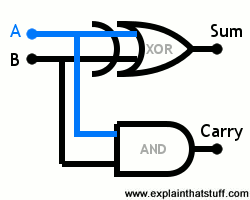You feed in two binary numbers you want to add on the two input lines A and B. They "travel" simultaneously to the inputs of the two logic gates—an XOR gate at the top and an AND gate underneath. The output from the XOR gate gives the sum of the two inputs, while the output from the AND gate tells us if we need to carry a 1. It'll be clearer what that means if we consider four possible calculations a half adder can do:

• If A and B both receive a zero, we're doing the sum 0 + 0 = 0. The XOR gate gives a zero if both its inputs are zero, and so does the AND gate. So the result of our sum is zero and the carry is zero.
• If A receives a zero and B receives a one, we're doing the sum 0 + 1 = 1. The XOR gate gives a one if either one (but not both) of its inputs is a one. The AND gate gives a one only if both of its inputs are one. So the result of our sum is one and the carry is zero.
• If A receives a one and B receives a zero, it's just the same as the previous example: the result of our sum is one and the carry is zero.
• Finally, if both A and B receive a one, we're doing the sum 1 + 1 = 2. Now the XOR gate gives a zero, while the AND gate gives a one. So the sum is zero and the carry is one, which means the overall result is 10 in binary or 2 in decimal.

Half adders can't really do any more than this, but if we put a few more logic gates together, we can make what's called a full adder circuit that does harder sums with bigger numbers. How does an adder work? That's beyond the scope of this introductory article, but you can find a few examples on the web pages below.

Unless you're doing a degree in electronics or computing, all you really need to know is that an adder is built from a number of AND, OR, and NOT logic gates contained inside chips that are connected together. We can use other patterns of logic gates to subtract, multiply (which can also be done by repeated addition), and do other kinds of calculations.

### Find out more

• How logic gates add 2+3: A nice diagram of an adder circuit from ZByte. [Archived via the Wayback Machine.]
• Adder (electronics): A more complex, more comprehensive, but harder to understand article on Wikipedia.

## Find out more

### On other websites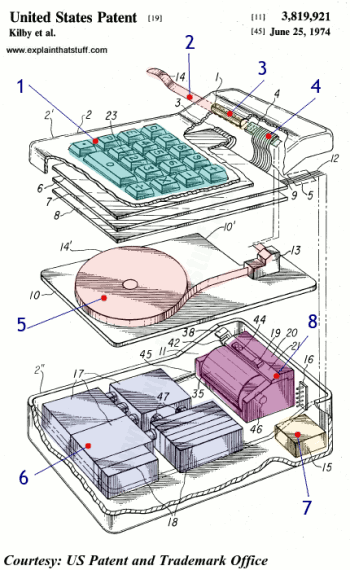Artwork: Who invented the pocket calculator? Jack Kilby and colleagues at Texas Instruments in a patent filed in 1972 and granted two years later. Here's how it worked: (1) You typed your sums into the keyboard and watched the answer appear, shortly afterward, on a printed paper tape (there was no display) at the top (2). A magnifying lens (3) helped you decipher the tiny numbers produced by the printer (4). Inside the case, we can see the paper tape that feeds the printer (5). Beneath it, there's a huge bank of batteries (6), a relatively tiny box full of electronics (7), and the thermal printer mechanism (8). Find out more in US Patent 3,819,921: Miniature electronic calculator. Artwork courtesy of US Patent and Trademark Office (coloring and large numbers added for clarity).

### Articles

#### History from the archives

• Those incredible new scientific pocket calculators by John Free. Popular Science, April 1974. This fascinating old article gives you a glimpse into how excited people were by programmable scientific calculators, which were the home computers of their day.
• Shakeout Time for Calculators by Nathaniel Nash. The New York Times, December 8, 1974. The Times breathlessly informs us that "one out of ten Americans now owns" a calculator!
• Calculators on a chip are here! by John Free. Popular Science, March 1973. Remember when calculators used to fill the windows of electronics stores?

### Patents

• US Patent 3,819,921: Miniature electronic calculator by Jack Kilby, Jerry Merryman, and James Van Tassel, Texas Instruments, granted 25 June 1974. Kilby, who co-invented integrated circuits with Robert Noyce, also pioneered the handheld calculator. Here's his original patent. If you really want to understand how calculators work, this is a great place to start.

Please do NOT copy our articles onto blogs and other websites

Articles from this website are registered at the US Copyright Office. Copying or otherwise using registered works without permission, removing this or other copyright notices, and/or infringing related rights could make you liable to severe civil or criminal penalties.